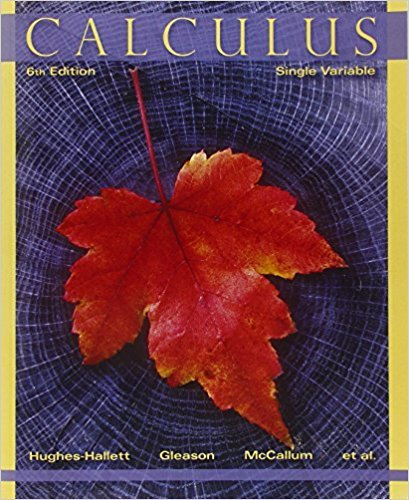×
×

# Solutions for Chapter 11.7: THE LOGISTIC MODEL## Full solutions for Calculus: Single Variable | 6th Edition

ISBN: 9780470888643Solutions for Chapter 11.7: THE LOGISTIC MODEL

Solutions for Chapter 11.7
4 5 0 313 Reviews
13
4
##### ISBN: 9780470888643

Calculus: Single Variable was written by and is associated to the ISBN: 9780470888643. Chapter 11.7: THE LOGISTIC MODEL includes 50 full step-by-step solutions. Since 50 problems in chapter 11.7: THE LOGISTIC MODEL have been answered, more than 33516 students have viewed full step-by-step solutions from this chapter. This textbook survival guide was created for the textbook: Calculus: Single Variable , edition: 6. This expansive textbook survival guide covers the following chapters and their solutions.

Key Calculus Terms and definitions covered in this textbook
• Augmented matrix

A matrix that represents a system of equations.

• Conjugate axis of a hyperbola

The line segment of length 2b that is perpendicular to the focal axis and has the center of the hyperbola as its midpoint

• Degree of a polynomial (function)

The largest exponent on the variable in any of the terms of the polynomial (function)

• Demand curve

p = g(x), where x represents demand and p represents price

• Imaginary unit

The complex number.

• Linear regression equation

Equation of a linear regression line

• Multiplication principle of counting

A principle used to find the number of ways an event can occur.

• n-set

A set of n objects.

• Nonsingular matrix

A square matrix with nonzero determinant

• Pie chart

See Circle graph.

The measure of an angle in radians, or, for a central angle, the ratio of the length of the intercepted arc tothe radius of the circle.

• Removable discontinuity at x = a

lim x:a- ƒ(x) = limx:a+ ƒ(x) but either the common limit is not equal ƒ(a) to ƒ(a) or is not defined

• Solve graphically

Use a graphical method, including use of a hand sketch or use of a grapher. When appropriate, the approximate solution should be confirmed algebraically

• Variance

The square of the standard deviation.

• Variation

See Power function.

• Velocity

A vector that specifies the motion of an object in terms of its speed and direction.

• Vertical line test

A test for determining whether a graph is a function.

• Weights

See Weighted mean.

• Xmin

The x-value of the left side of the viewing window,.

• Zoom out

A procedure of a graphing utility used to view more of the coordinate plane (used, for example, to find theend behavior of a function).

×